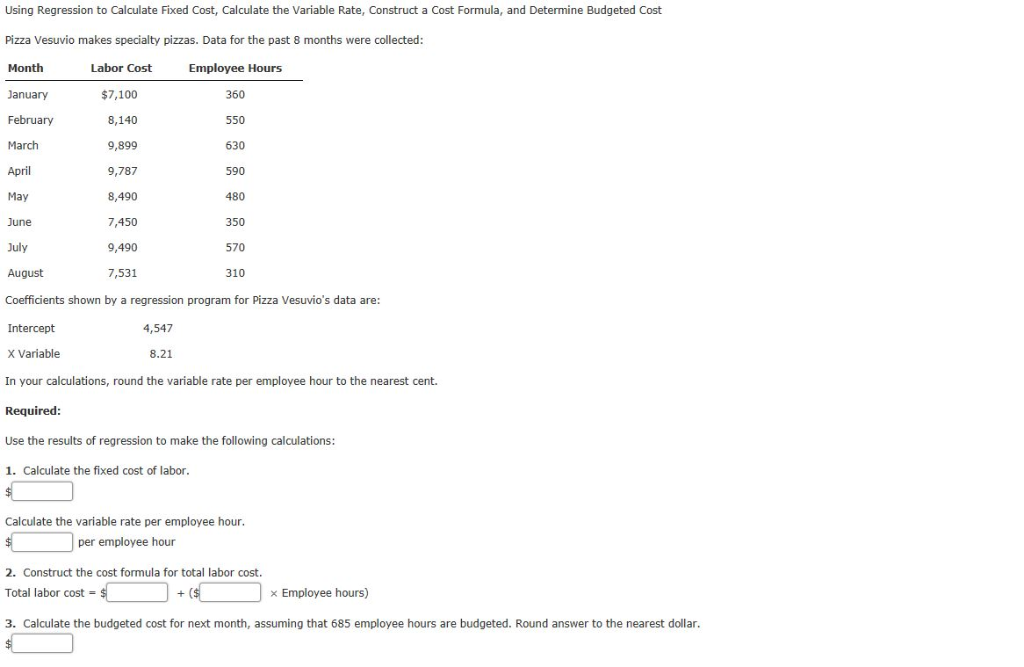### Create an Account

Home / Questions / Using Regression to Calculate Fixed Cost, Calculate the Variable Rate, Construct a Cost Formula, and

# Using Regression to Calculate Fixed Cost, Calculate the Variable Rate, Construct a Cost Formula, andUsing Regression to Calculate Fixed Cost, Calculate the Variable Rate, Construct a Cost Formula, and Determine Budgeted Cost Pizza Vesuvio makes specialty pizzas. Data for the past 8 months were collected: Month Labor Cost Employee Hours January \$7,100 360 February 8,140 550 March 9,899 630 April 9,787 590 May 8,490 480 350 June 7,450 July 9,490 570 7,531 August 310 Coefficients shown by a regression program for Pizza Vesuvio&#39;s data are: Intercept 4,547 x Variable 8.21 In your calculations, round the variable rate per employee hour to the nearest cent. Required: Use the results of regression to make the following calculations: 1. Calculate the fixed cost of labor. Calculate the variable rate per employee hour. per employee hour 2. Construct the cost formula for total labor cost. Total labor cost \$ (\$ Employee hours) 3. Calculate the budgeted cost for next month, assuming that 685 employee hours are budgeted. Round answer to the nearest dollar.

Apr 10 2020 View more View LessSubscribe To Get Solution